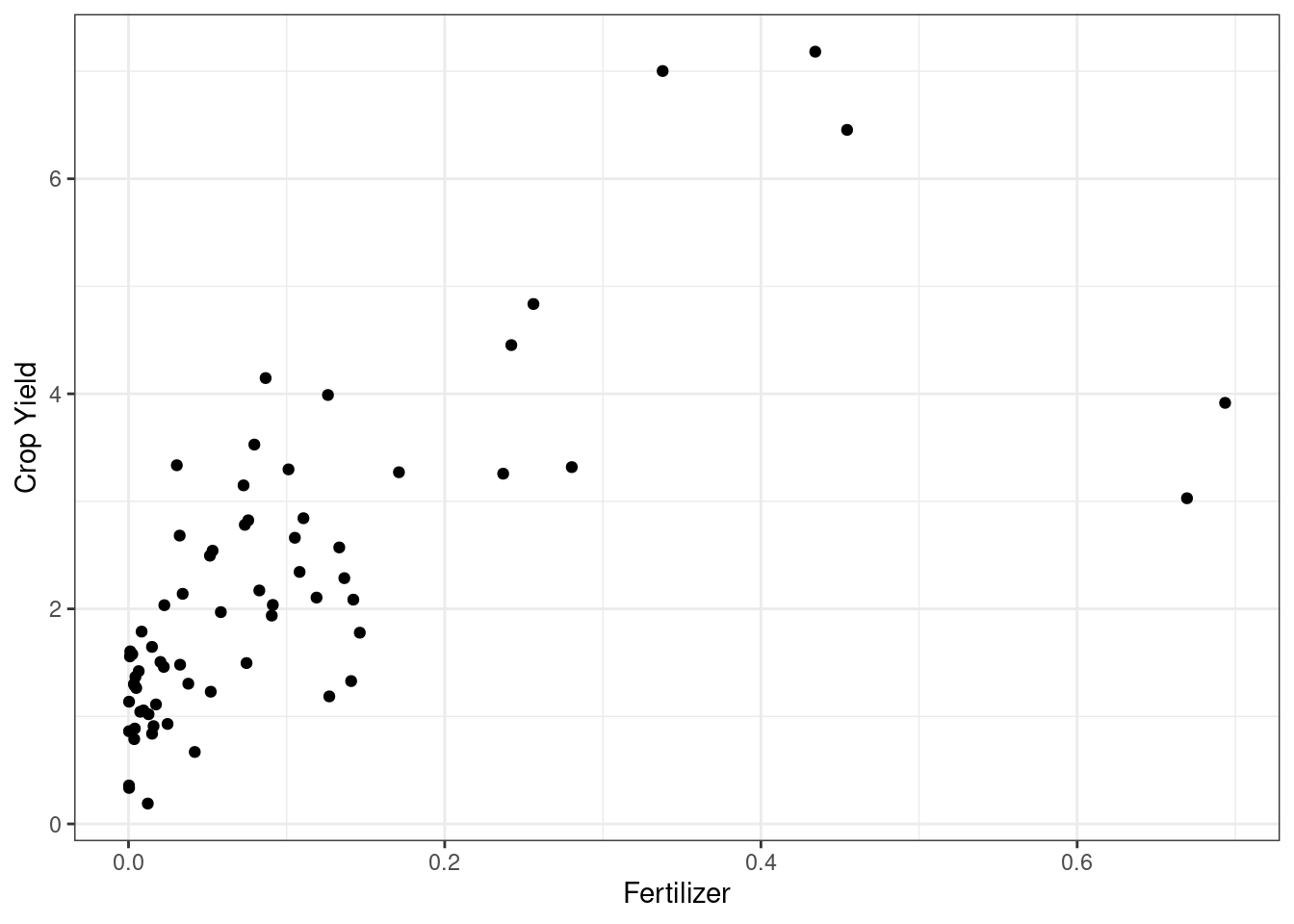# Ch. 3, Coding Question 2

# load data

library(ggplot2)

# make scatter plot
ggplot(data=fertilizer_2000,
mapping=aes(x=avfert, y=avyield)) +
geom_point() +
ylab("Crop Yield") +
xlab("Fertilizer") +
theme_bw()It seems like countries with higher fertilizer usage tend to have higher crop yields.

# Ch. 3, Coding Question 3

# load data
library(Ecdat)
data("Airq", package="Ecdat")

# a) estimate mean rainfall
ybar <- mean(Airq$rain) ybar ##  36.078 # b) standard error V <- var(Airq$rain)
n <- nrow(Airq)
se <- sqrt(V)/sqrt(n)
se
##  2.462628
# c) t-statistic
h0 <- 25
t <- (ybar-h0)/se
t
##  4.498446

Since $$|t| > 1.96$$, we would reject $$H_0$$ at the 5% significance level.

# d) p-value
pval <- 2*pnorm(-abs(t))
pval
##  6.845183e-06

There is virtually a 0 percent chance of getting a t-statistic this large in absolute value if the null hypotheses were true.

# e) confidence interval
ciL <- ybar - 1.96*se
ciU <- ybar + 1.96*se
paste0("[",round(ciL,3),", ", round(ciU,3), "]")
##  "[31.251, 40.905]"
# f) summary statistics
library(modelsummary)
datasummary_balance(~coas, Airq)
no (N=9)
yes (N=21)
Mean Std. Dev. Mean Std. Dev. Diff. in Means Std. Error
airq 125.3 10.5 95.9 28.7 −29.5 7.2
vala 4118.2 5909.8 4218.6 4136.7 100.4 2166.9
rain 32.3 7.6 37.7 15.2 5.4 4.2
dens 1706.4 3014.6 1738.1 2821.2 31.7 1178.5
medi 6290.3 10065.4 10842.2 13396.8 4551.9 4450.1

# Ch. 3, Extra Question 5

Consistency is a large sample property for an estimator. It says that, if we have a large sample, then our estimator should be close to the population quantity that we are trying to estimate.

An unbiased estimator is one that, if we could repeatedly draw samples of size $$n$$ ($$n$$ could be large or small here) and re-estimate the parameter of interest, then on average (across samples), we our estimate would be equal to the population parameter that we are trying to estimate.

# Ch. 3, Extra Question 6

No, an unbiased estimator does not necessarily have to be consistent. Consider estimating $$\mathbb{E}[Y]$$ by $$Y_1$$ (i.e., using the value of $$Y$$ for the first observation in the data). This estimate of $$\mathbb{E}[Y]$$ is unbiased (since $$\mathbb{E}[Y_1] = \mathbb{E}[Y])$$, but it is not consistent (because it does not even depend on the sample size at all).

# Ch. 3, Extra Question 7

No, consistent estimators can be biased. Consider estimating $$\mathbb{E}[Y]$$ by $$\bar{Y} + \frac{c}{n}$$ where $$c$$ is a constant. This is consistent because $$\bar{Y} \rightarrow \mathbb{E}[Y]$$ as $$n \rightarrow \infty$$ (by the law of large numbers) and $$\frac{c}{n} \rightarrow 0$$ as $$n \rightarrow \infty$$ (because it is a constant divided by a number that is going to infinity). However, notice that

\begin{aligned} \mathbb{E}\left[\bar{Y} + \frac{c}{n} \right] &= \mathbb{E}[Y] + \frac{c}{n} \\ & \neq \mathbb{E}[Y] \end{aligned} so, for any fixed sample size $$n$$, $$\bar{Y} + \frac{c}{n}$$ is biased for $$\mathbb{E}[Y]$$.

# Ch. 3, Extra Question 8

1. Since $$n$$ grows faster than $$\sqrt{n}$$, $$n \left(\frac{1}{n}\sum_{i=1}^n (Y_i - \mathbb{E}[Y])\right)$$ diverges (i.e., the absolute value goes to infinity as $$n \rightarrow \infty$$)

2. Since $$n^{1/3}$$ grows slower than $$\sqrt{n}$$, $$n^{1/3} \left(\frac{1}{n}\sum_{i=1}^n (Y_i - \mathbb{E}[Y])\right)$$ converges to 0 as $$n \rightarrow \infty$$